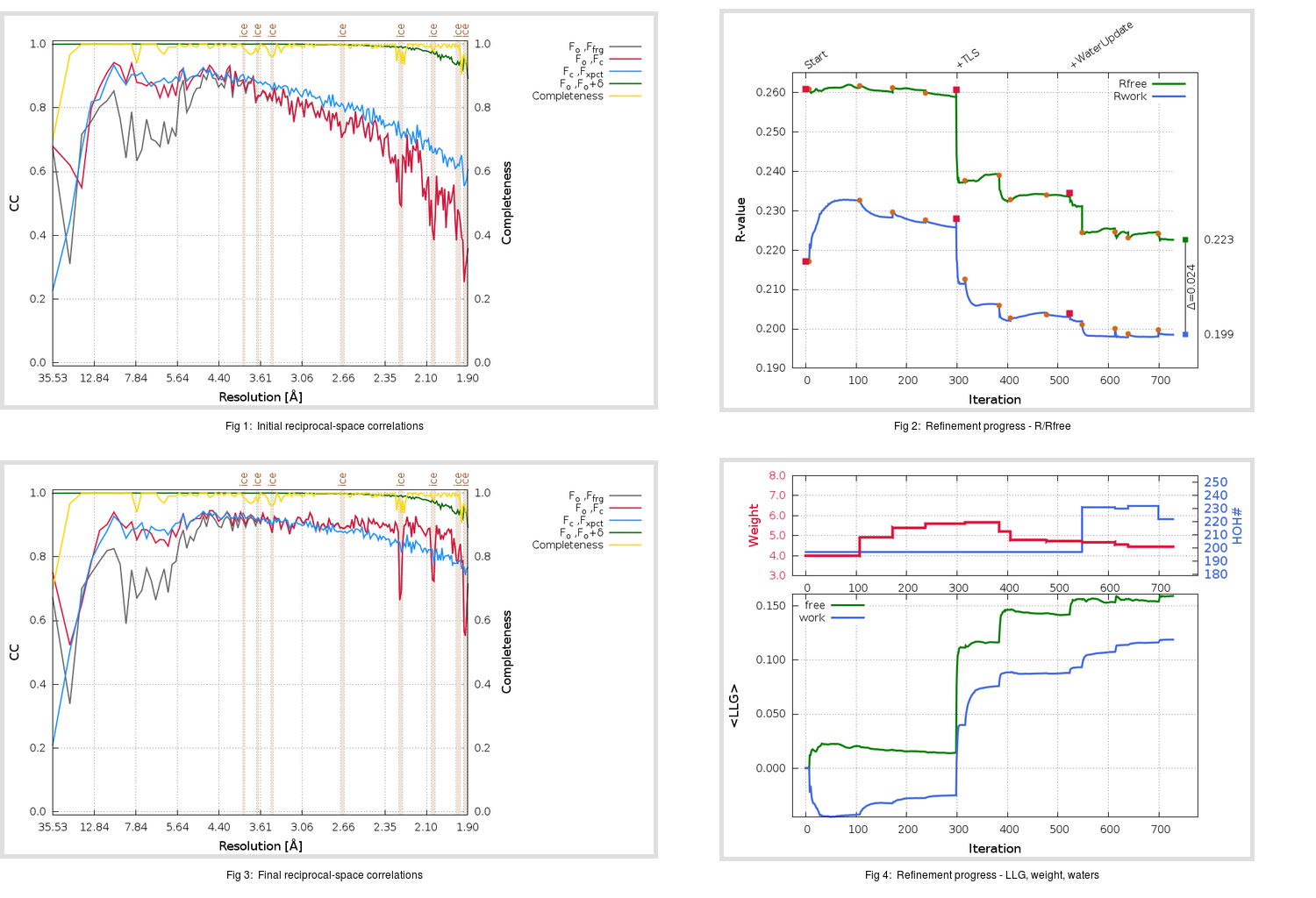Content:

```    Diffraction limits & principal axes of ellipsoid fitted to diffraction cut-off surface:
1.862         0.9043   0.0000   0.4268       0.995 a* + 0.102 c*
1.912         0.0000   1.0000   0.0000       b*
1.818        -0.4268   0.0000   0.9043      -0.662 a* + 0.750 c*
```

## Deposited

` `
 Date deposited Date data collection Resolution R, Rfree 20200301 20190321 1.90 0.2062 0.2576

Molprobity (CCP4 7.0 version) summary:

```Ramachandran outliers =   0.33 %
favored =  96.71 %
Rotamer outliers      =   3.41 %
C-beta deviations     =     0
Clashscore            =   3.78
RMS(bonds)            =   0.0145
RMS(angles)           =   1.94
MolProbity score      =   1.78
Resolution            =   1.90
R-work                =   0.2062
R-free                =   0.2576
```

```Number of waters      =   197

<B> (all atoms) =   52.35 ( sd =   14.80 ) for       2622 non-hydrogen atoms
<B>   (protein) =   51.62 ( sd =   14.96 ) for       2379 non-hydrogen atoms
<B>     (water) =   57.53 ( sd =   10.20 ) for        197 non-hydrogen atoms
<B>    (others) =   71.40 ( sd =   21.57 ) for         46 non-hydrogen atoms

B min/max       (all non-hydrogen atoms) =   27.14 /  113.43
B min/max   (protein non-hydrogen atoms) =   27.14 /  113.43
B min/max     (water non-hydrogen atoms) =   33.42 /   84.06
B min/max     (other non-hydrogen atoms) =   43.35 /  112.98
```

## BUSTER (re-)refinement

` `

Molprobity (CCP4 7.0 version) summary:

```Ramachandran outliers =   0.00 %
favored =  98.03 %
Rotamer outliers      =   3.03 %
C-beta deviations     =     1
Clashscore            =   2.10
RMS(bonds)            =   0.0113
RMS(angles)           =   1.56
MolProbity score      =   1.35
Resolution            =   1.90
R-work                =   0.1988
R-free                =   0.2228
```

```Number of waters      =   222

<B> (all atoms) =   50.45 ( sd =   13.60 ) for       2647 non-hydrogen atoms
<B>   (protein) =   49.32 ( sd =   13.16 ) for       2379 non-hydrogen atoms
<B>     (water) =   60.20 ( sd =   12.91 ) for        222 non-hydrogen atoms
<B>    (others) =   64.44 ( sd =   22.62 ) for         46 non-hydrogen atoms

B min/max       (all non-hydrogen atoms) =   26.65 /  124.37
B min/max   (protein non-hydrogen atoms) =   26.65 /   99.93
B min/max     (water non-hydrogen atoms) =   28.65 /  124.37
B min/max     (other non-hydrogen atoms) =   47.53 /  108.87
```

Refinement progression:Results:

` `
 File Remark 6Y7M_aB_refine.01_03_refine.pdb.gz exact refinement commands are in header 6Y7M_aB_refine.01_03_refine.mtz.gz including original deposited data and several re-refinement map coefficients 6Y7M_aB_refine.01_03_BUSTER_model.cif.gz including any non-standard compound restraints 6Y7M_aB_refine.01_03_BUSTER_refln.cif.gz